Latest Banking jobs   »

# Quantitative Aptitude Quiz For RBI Grade B/ ECGC PO/ SIDBI Grade A Prelims 2022- 13th May

Directions (1-5): The following questions are accompanied by two statements (I) and (II). You have to determine which statements(s) is/are sufficient/necessary to answer the questions.

Q1. Find the value of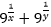?
I. The difference of inverse of x & y is 8/3.
II. the multiplication of x & y is 3.
(a) Only I
(b) Only II
(c) Either I or II
(d) Only I and II
(e) None of the above

Q2. How many women are required to complete the work in 12 days.
I. Efficiency of men are ⅔ rd of efficiency of women.
II. 16 men can complete the same work in 10 days.
(a) Only I
(b) Only II
(c) Either I or II
(d) Only I and II
(e) None of the above

Q3. In the triangle ABC. Find ∠ABD ?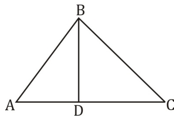I. Given that BC = CD.
II. ∠ABC – ∠BAC = 30°
(a) Only I
(b) Only II
(c) Either I or II
(d) Only I and II
(e) None of the above

Q4. What is the C.I. on a sum at the end of 3 years ?
I. C.I. at the end of two years is Rs. 110.
II. Difference between CI and SI at the end of two year is Rs. 100 and rate percent is 10%.
(a) Only I
(b) Only II
(c) Either I or II
(d) Only I and II
(e) None of the above

Q5. Two trains X and Y starts from point A and B respectively towards each other. What is the distance between them when they start ?
I. When both trains cross each other, time taken by train X to reach B is twice the time taken by train Y to reach A.
II. Distance between them after 60 min. is 800 km.
(a) Only I
(b) Only II
(c) Either I or II
(d) Only I and II
(e) None of the above

Directions (6-10): The following questions are accompanied by two statements (A) and (B). You have to determine which statement(s) is/are sufficient /necessary to answer the questions

(a) if the Statement ‘A’ alone is sufficient to answer the question but the Statement ‘B’ alone is not sufficient
(b) if the Statement ‘B’ alone is sufficient to answer the question but the Statement ‘A’ alone is not sufficient
(c) if both Statement ‘A’ and ‘B’ together are needed to answer the question
(d) if either the Statement ‘A’ alone or Statement ‘B’ alone is sufficient to answer the question
(e) if you cannot get the answer from both the Statements together

Q6. Find the value of 8y² (y + 3x) + 8x² (x + 3y)
A) Product of x and y is equal to 6
B) Sum of inverse of x and y is equals to 5/6

Q7. If X, Y and Z together can complete the work in 8 days, find in how many days Y alone can complete the work?
A) X and Y together can complete the work in 12 days while Z is 25% less efficient than ‘X’.
B) Z is 50% more efficient than ‘Y’ who is 100% less efficient than ‘X’.

Q8. What will be the speed of boat if A is the starting point and B is the destination point?
A) Man go to a point B and come back to initial point A in total 12 hours while the ratio of speed of boat to speed of stream is 3 : 2.
B) Man reached at mid-point of A and B in 5 hour and then go to point B and come back to mid-point of A and B in total 6 hours while speed of stream is 10 km/hr.

Q9. Three numbers are co-prime to each other. Find the sum of these numbers?
A) Product of first two number is 286 while the product of last two numbers is 470.
B) Sum of first two number is 35 while the sum of last two number is 57.

Q10. Find the sum of digits of given two-digit number?
A) If we interchange the numbers then the new number formed is greater than the original number by 9.
B) If we interchange the number then product of new number and original number is 1462.

Direction (11-15): In the given questions, two quantities are given, one as ‘Quantity 1’ and another as ‘Quantity 2’. You have to determine relationship between two quantities and choose the appropriate option:
(a) Quantity 1 > Quantity 2
(b) Quantity 1 ≥ Quantity 2
(c) Quantity 2 > Quantity 1
(d) Quantity 2 ≥ Quantity 1
(e) Quantity 1 = Quantity 2 or Relation cannot be established

Q11. Quantity 1: Find the speed of train (in m/s) if the train takes 20 sec to completely cross another train coming from opposite direction of twice the length & speed both as that of first train. The first train can cross a platform 100 m long in 30 sec.
Quantity 2: Find the speed of train (in m/s) if the train overtakes another train running at a speed of 20 m/s in 70 sec while the latter train can cross a platform of same length in 25 sec. Length of first train is 60% less than that of latter train. (latter train is faster)

Q12. Quantity 1: Find the number of blue balls in the bag if the probability of getting a red ball when 3 balls are drawn at random from the bag having 15 balls is 45/91. If there would be no blue balls, this probability would be 5/11. The bag contains red, blue & green color balls and the blue balls are minimum in the bag.
Quantity 2: Find the number of blue balls in the bag if the bag contains ‘x’ red balls & ‘y’ blue balls such that x²-3x-10=0 and probability of drawing 2 red balls from the bag at random is 2/9.

Q13. Quantity 1: Find the value of (a+b-c)² if(a+b)²=25=b²+c²; a < b < c & a, b, c ∈ N
Quantity 2: Find the value of (a+b-c)² if a :b=1:1; a is 40% less than c and average of b & c is 4.

Q14. Quantity 1: In what time (min) the tank will be filled completely if all the pipes are opened simultaneously. There are 3 pipes of which 2 are inlet and other is outlet. If there is only 1 inlet (which is the most efficient), then tank will be filled by both the pipes in 30 min. The inlet pipes alone take 10 & 12 min respectively to fill the tank. All pipes are opened till tank is filled.
Quantity 2: In what time (min) the tank will be filled completely if all the pipes are opened simultaneously. Pipe A, B & C alone can fill the tank in 15, 12 & 10 min respectively. Pipes are opened in a pair for a minute & no pipe works for more than 2 min continuously. (consider minimum time taken)

Q15. Quantity 1: Curved surface area of sphere is 616 sq.m. Find curved surface area of cylinder (sq.m.) if the sphere is melted to form a cylinder of height 28/3 m.
Quantity 2: Curved surface area of sphere is 616 sq.m. Find curved surface area of cylinder (sq.m.) if the cylinder can completely fit inside the sphere such that top & bottom of cylinder lies on surface of sphere. Radius of cylinder = 7 m.

Solutions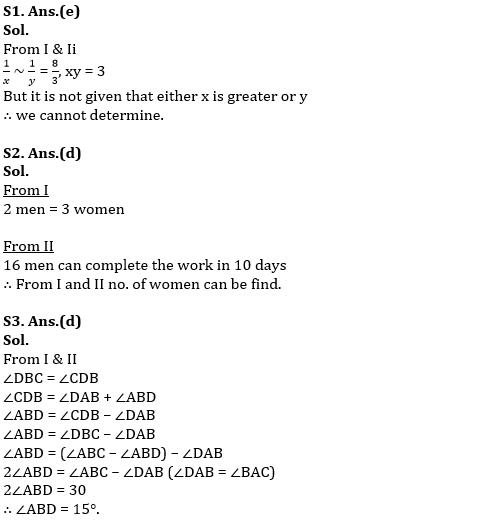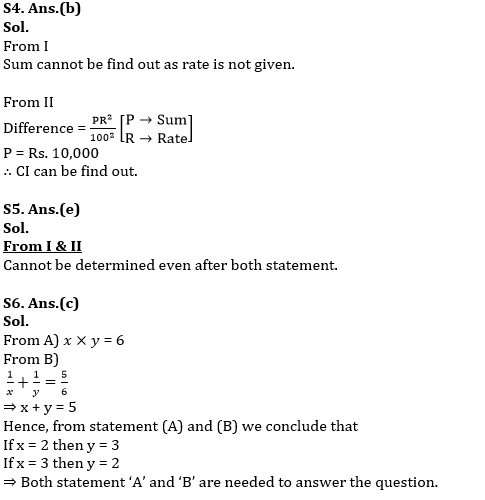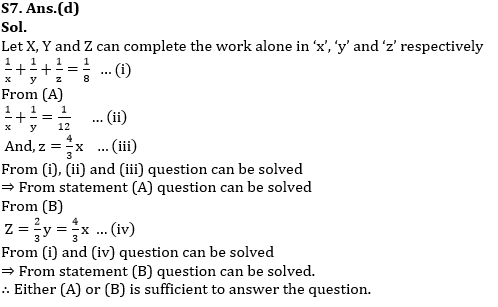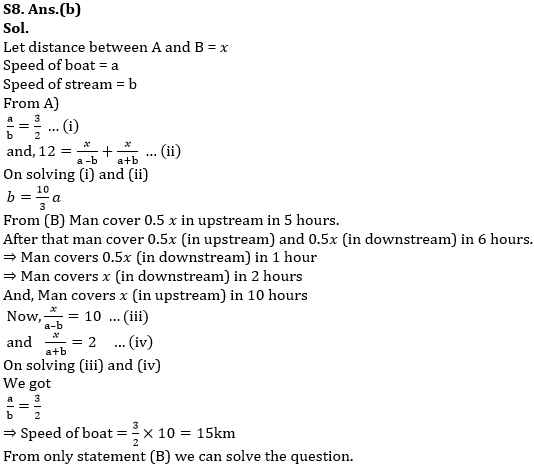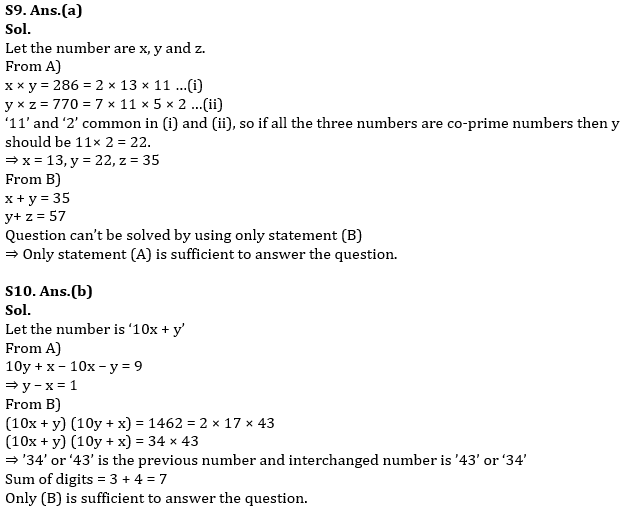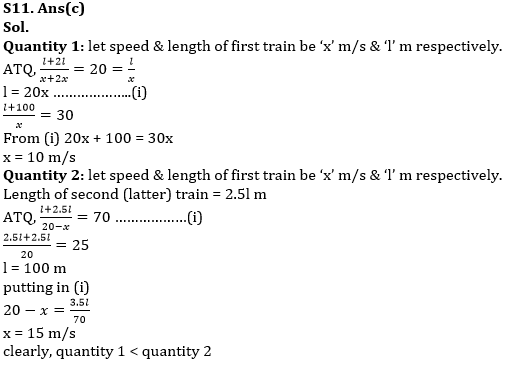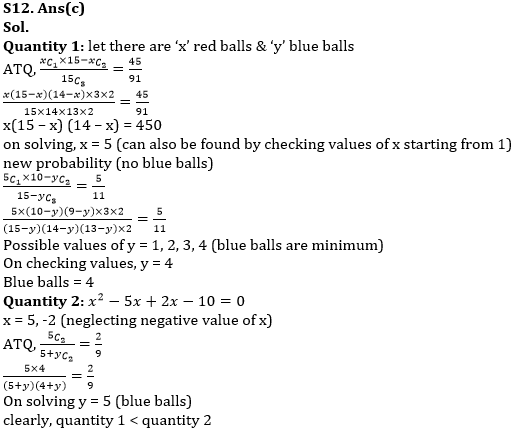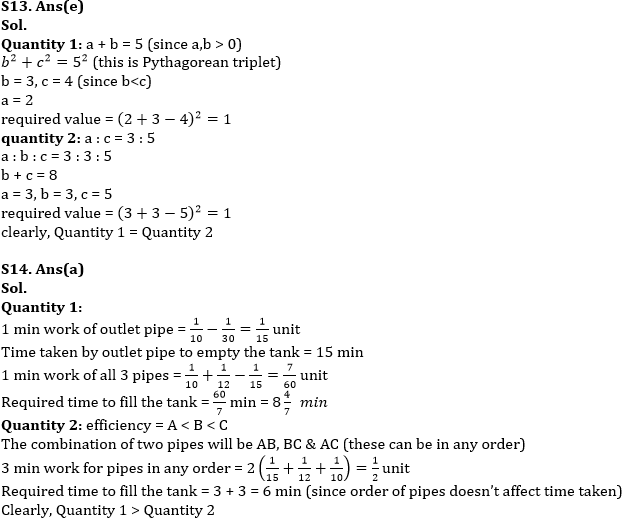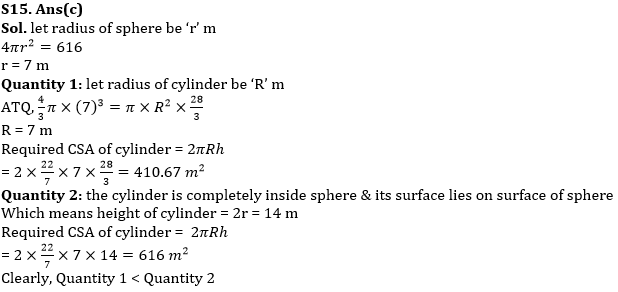#### Congratulations!Union Budget 2023-24: Free PDF